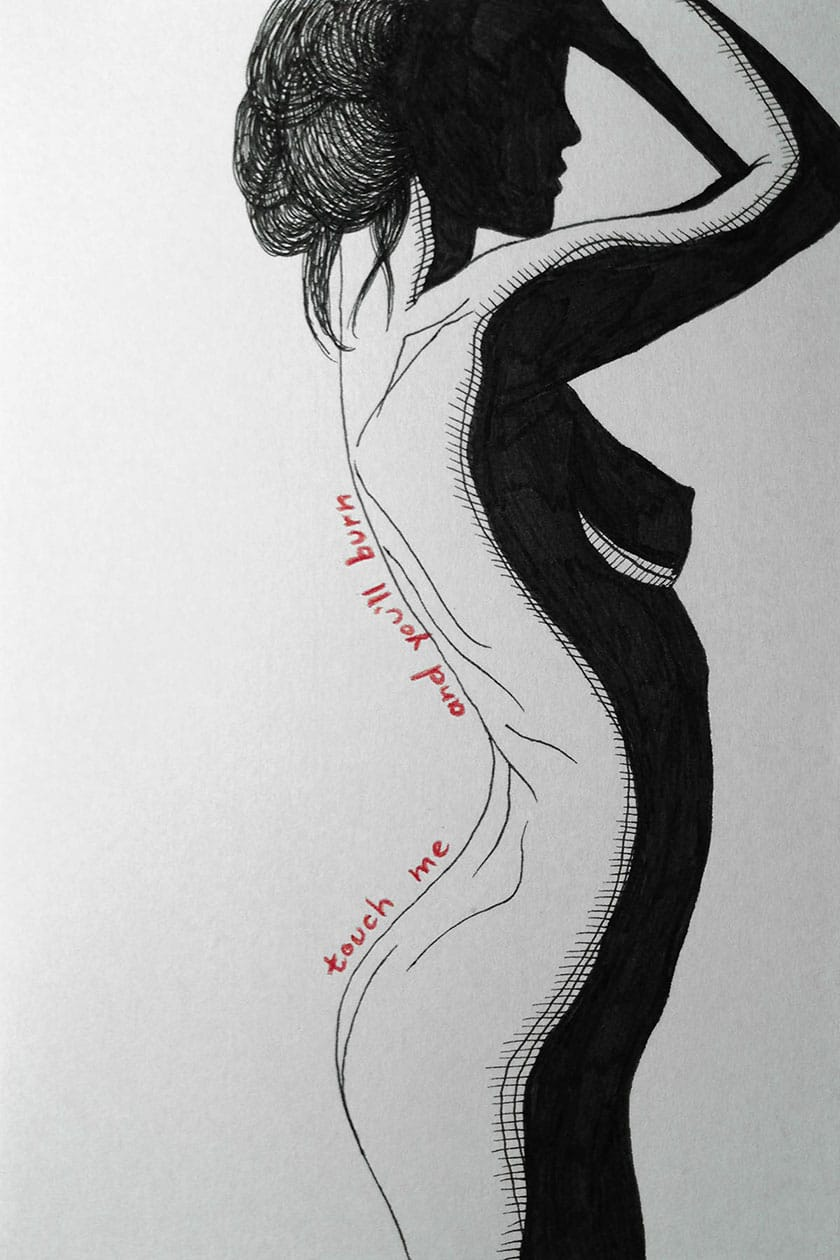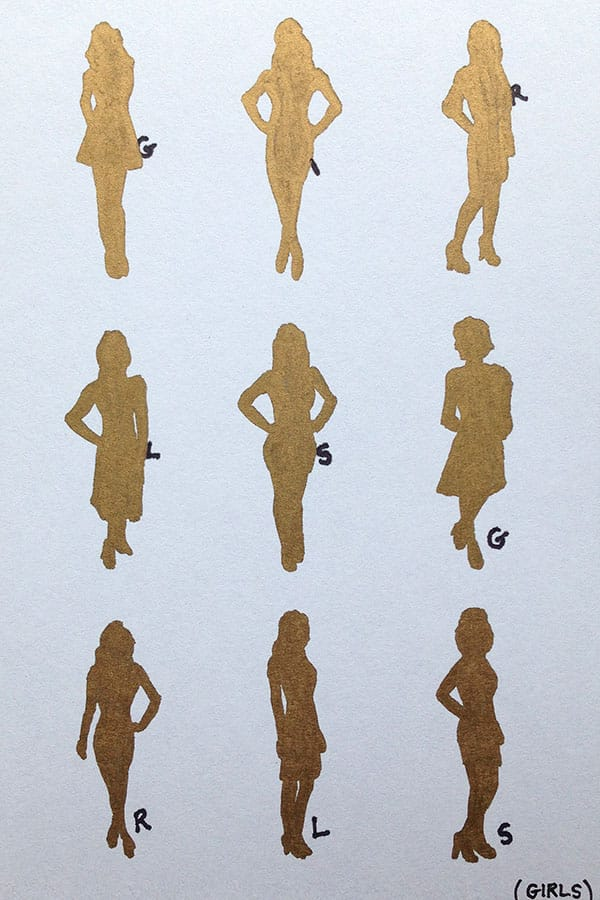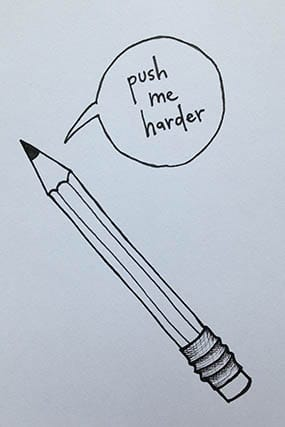# Project Euler: #137, Fibonacci golden nuggets

Thing practiced: math

Tools used: A Friendly Introduction to Number Theory, Python

“m tired of girls, I want to see more math.”
—no one ever

Problem:

Consider the infinite polynomial series

$${A_F}(x) = x{F_1} + {x^2}{F_2} + {x^3}{F_3} + \ldots \tag{1}$$

where Fk is the kth term in the Fibonacci sequence (1, 1, 2, 3, 5, 8, ...).

For every case where x is rational and AF(x) is a positive integer, we call AF(x) a golden nugget, because these are increasingly rare – for example, the 10th golden nugget is 74,049,690.

What is the 15th golden nugget?

Solution:

Infinite series are unwieldy, so first we replace (1) with a closed-form version (derived here):

$${A_F}(x) = \frac{x}{1 - x - {x^2}} \tag{2}$$

We need AF(x) to be a positive integer, which we’ll call n. So, rearranging (2), we get:

$${A_F}(x) = n = \frac{x}{1 - x - {x^2}}$$ $$n{x^2} + (n + 1)x - n = 0 \tag{3}$$

This is a standard quadratic equation with solutions

$$x = \frac{-(n + 1) \pm \sqrt{(n + 1)^2 + 4n^2}}{2n} \tag{4}$$

As long as the square root portion of (4) is an integer, x will be rational. We can solve for this case by calling this portion m (making the discriminant m2):

$$m^2 = (n + 1)^2 + 4{n^2} = 5{n^2} + 2n + 1$$

and doing some algebra we get:

$$5m^2 = 25n^2 + 10n + 1 + 4 = (5n + 1)^2 + 4$$ $$(5n + 1)^2 - 5m^2 = -4 \tag{5}$$

Now, if we substitute p = 5n + 1, then (5) becomes a Pell-like equation:

$$p^2 - 5m^2 = -4 \tag{6}$$

This means that we can find solutions to (6) by finding solutions to the corresponding unit-form Pell equation x2 - 5y2 = 1 and transforming them.

To compute the solutions, we use the technique described here. We first identify the fundamental solutions to (6). Then, we transform these into solutions of the unit-form equation by the identity (pi2 - 5mi2)(xi2 - 5yi2) = -4. From these, we can generate subsequent solutions, then transform them back into solutions to (6) by the same identity. Finally, we re-substitute our original n = AF(x) back in to find the golden nuggets.

This gives us the answer: 1,120,149,658,760.

# Early morning doodles

Thing practiced: drawing

Tools used: index cards, pen, colored pencil, phone# Waiting-around doodles

Thing practiced: handheld drawing

Tools used: free minutes, index cards, Sharpie®s